Homework Help Question & Answers

# 1 point) Integratef(x, y, z) 6xz over the region in the first octant (x,y, z 0) above the parabol...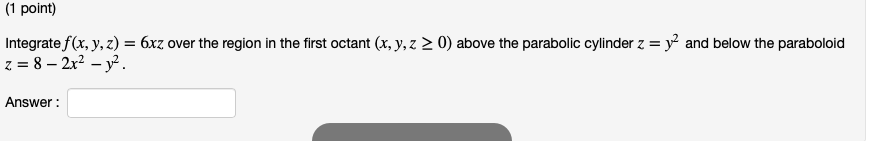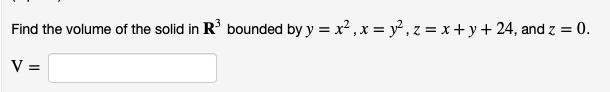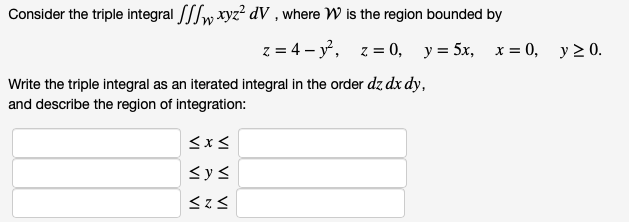Please try helping with all three questions.......please

1 point) Integratef(x, y, z) 6xz over the region in the first octant (x,y, z 0) above the parabolic cylinder z = y2 and below the paraboloid Answer
Find the volume of the solid in R3 bounded by y-x2 , x-уг, z-x + y + 24, and Z-0.
Consider the triple integral fsPw xyz2 dV, where W is the region bounded by Write the triple integral as an iterated integral in the order dz dx dy and describe the region of integration: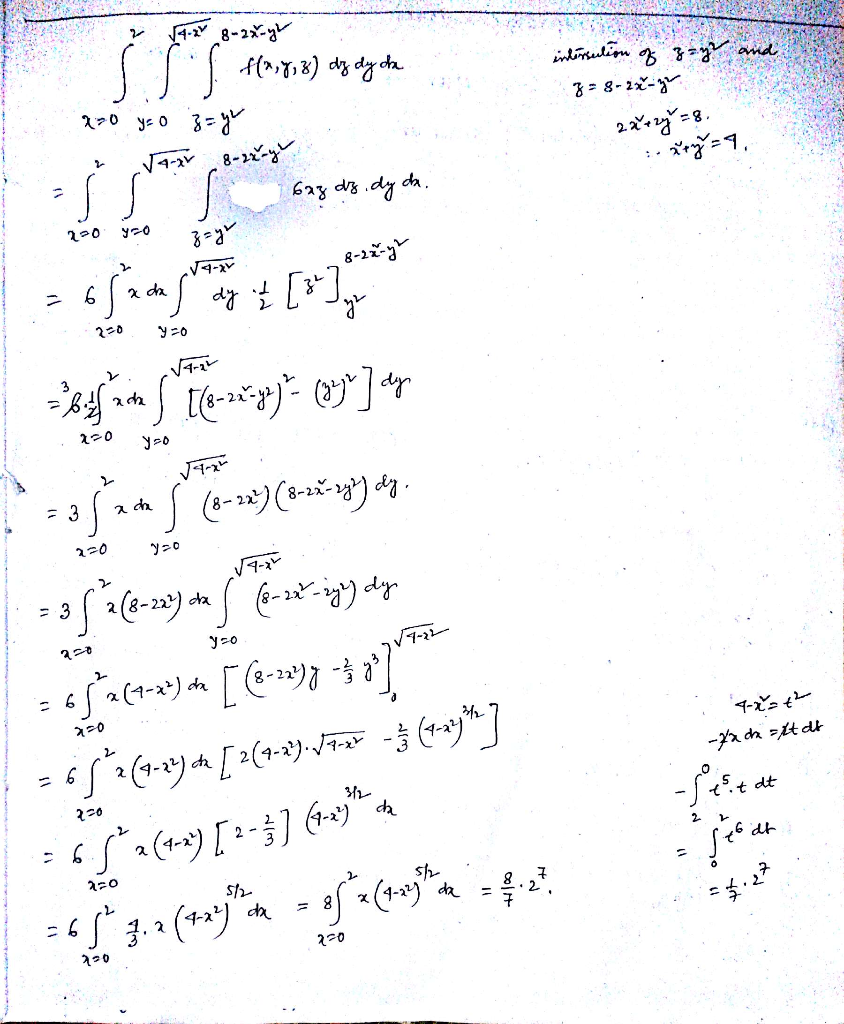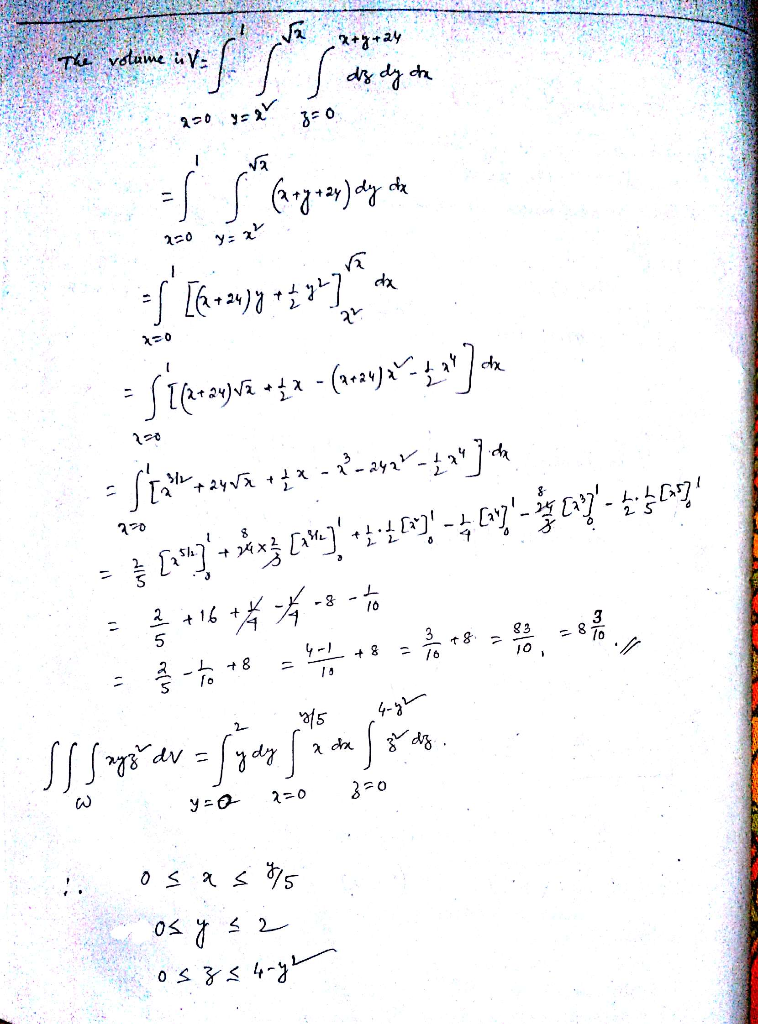##### Add Answer of: 1 point) Integratef(x, y, z) 6xz over the region in the first octant (x,y, z 0) above the parabol...
More Homework Help Questions Additional questions in this topic.

• #### Let D be a region in the (a, y)-plane. If a, b, e>0, let Sa be the part of the hyperbolic parabol...

Need Online Homework Help?

Get FREE EXPERT Answers
WITHIN MINUTES
Related Questions